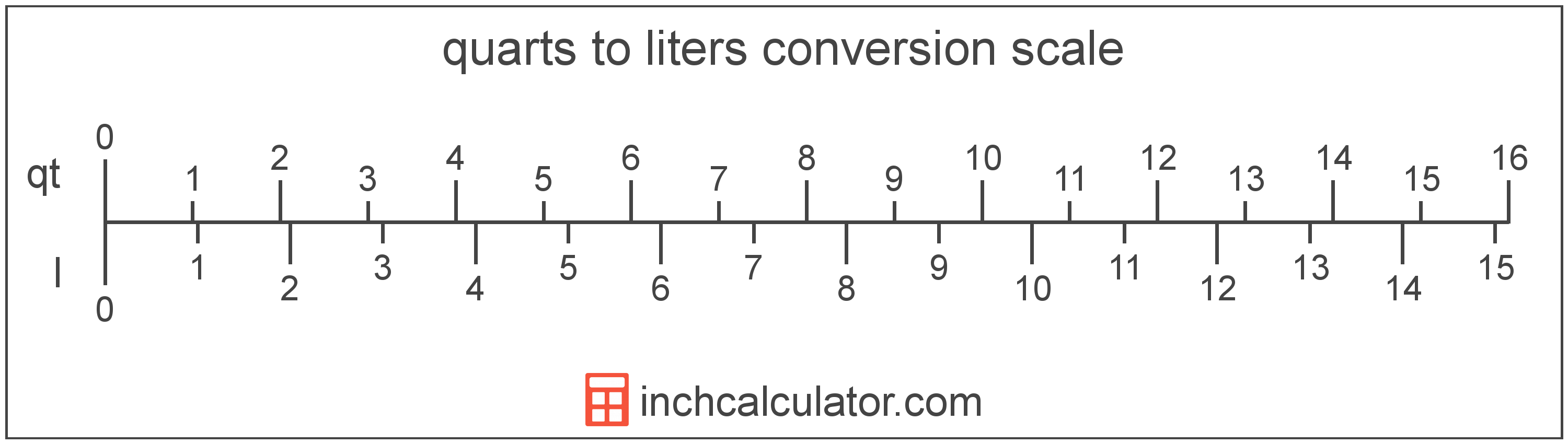# Quarts to Liters Converter

Enter the volume in quarts below to get the value converted to liters.

Results in Liters:1 qt = 0.946353 L

Do you want to convert liters to quarts?

## How to Convert Quarts to Liters

To convert a measurement in quarts to a measurement in liters, multiply the volume by the following conversion ratio: 0.946353 liters/quart.

Since one quart is equal to 0.946353 liters, you can use this simple formula to convert:

liters = quarts × 0.946353

The volume in liters is equal to the volume in quarts multiplied by 0.946353.

For example, here's how to convert 5 quarts to liters using the formula above.
liters = (5 qt × 0.946353) = 4.731765 L### How Many Liters Are in a Quart?

There are 0.946353 liters in a quart, which is why we use this value in the formula above.

1 qt = 0.946353 L

## What is a Quart?

The US liquid quart is a unit of fluid volume equal to one fourth of a gallon, two pints, or four cups.

The liquid quart should not be confused with the dry quart (US) or the imperial quart, which are different units. However, when someone refers to a volume in quarts, they typically mean the US liquid quart.

The quart is a US customary unit of volume. Quarts can be abbreviated as qt; for example, 1 quart can be written as 1 qt.

## What is a Liter?

A liter is a unit of volume equal to 1,000 cubic centimeters or 0.264172 US gallons. The liter is a special name defined for the cubic decimeter and is exactly equal to the volume of one cubic decimeter (1 decimeter is 1/10 of a meter, or 10 centimeters).

The liter is an SI accepted unit for volume for use with the metric system. A liter is sometimes also referred to as a litre. Liters can be abbreviated as L, and are also sometimes abbreviated as l or . For example, 1 liter can be written as 1 L, 1 l, or 1 ℓ.

## Quart to Liter Conversion Table

Table showing various quart measurements converted to liters.
Quarts Liters
1 qt 0.946353 L
2 qt 1.8927 L
3 qt 2.8391 L
4 qt 3.7854 L
5 qt 4.7318 L
6 qt 5.6781 L
7 qt 6.6245 L
8 qt 7.5708 L
9 qt 8.5172 L
10 qt 9.4635 L
11 qt 10.41 L
12 qt 11.36 L
13 qt 12.3 L
14 qt 13.25 L
15 qt 14.2 L
16 qt 15.14 L
17 qt 16.09 L
18 qt 17.03 L
19 qt 17.98 L
20 qt 18.93 L
21 qt 19.87 L
22 qt 20.82 L
23 qt 21.77 L
24 qt 22.71 L
25 qt 23.66 L
26 qt 24.61 L
27 qt 25.55 L
28 qt 26.5 L
29 qt 27.44 L
30 qt 28.39 L
31 qt 29.34 L
32 qt 30.28 L
33 qt 31.23 L
34 qt 32.18 L
35 qt 33.12 L
36 qt 34.07 L
37 qt 35.02 L
38 qt 35.96 L
39 qt 36.91 L
40 qt 37.85 L

## References

1. National Institute of Standards and Technology, Units outside the SI, https://physics.nist.gov/cuu/Units/outside.html